## Gram-Schmidt Orthonormalization

A procedure which takes a nonorthogonal set of Linearly Independent functions and constructs an Orthogonal Basis over an arbitrary interval with respect to an arbitrary Weighting Function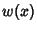. Given an original set of linearly independent functions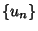, let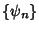denote the orthogonalized (but not normalized) functions and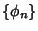the orthonormalized functions.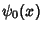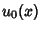(1)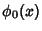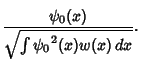(2)

Take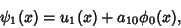(3)

where we require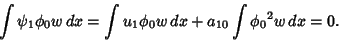(4)

By definition,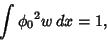(5)

so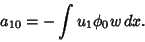(6)

The first orthogonalized function is therefore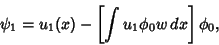(7)

and the corresponding normalized function is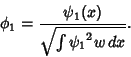(8)

By mathematical induction, it follows that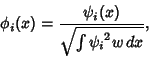(9)

where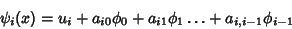(10)

and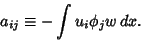(11)

If the functions are normalized to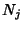instead of 1, then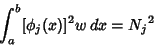(12)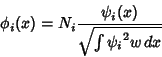(13)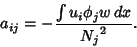(14)

Orthogonal Polynomials are especially easy to generate using Gram-Schmidt Orthonormalization. Use the notation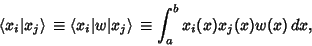(15)

whereis a Weighting Function, and define the first few Polynomials,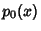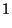(16)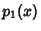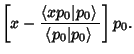(17)

As defined,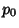and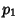are Orthogonal Polynomials, as can be seen from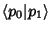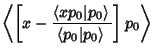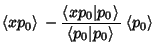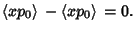(18)

Now use the Recurrence Relation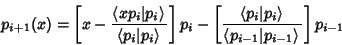(19)

to construct all higher order Polynomials.

To verify that this procedure does indeed produce Orthogonal Polynomials, examine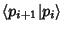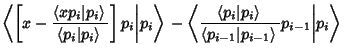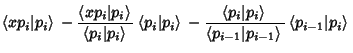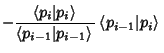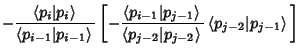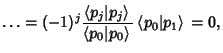(20)

since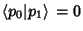. Therefore, all the Polynomials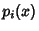are orthogonal. Many common Orthogonal Polynomials of mathematical physics can be generated in this manner. However, the process is numerically unstable (Golub and van Loan 1989).

Arfken, G. Gram-Schmidt Orthogonalization.'' §9.3 in Mathematical Methods for Physicists, 3rd ed. Orlando, FL: Academic Press, pp. 516-520, 1985.